# NCERT Solutions for Class 8 Maths Chapter 4 Practical Geometry| PDF Download

Here, you can find NCERT Solutions of Chapter 4 Practical Geometry Class 8 that will help you in understanding the topics given in the chapter. Chapter 4 Practical Geometry NCERT Solutions PDF Download is also available here which will develop your skills and improving marks in the examinations. These Chapter 4 NCERT Solutions are accurate and detailed and as per the latest syllabus released by CBSE.

Through the help of NCERT Solutions for Class 8, you can also complete your homework on time and able to solve the difficult problems in a exercise. It will improve the learning behaviour among students and help in solving a question efficiently.Page No: 60

Exercise 4.1

LI = 4 cm, IF = 3 cm, TL = 2.5 cm, LF = 4.5 cm, IT = 4 cm

OL = 7.5 cm, GL = 6 cm, GD = 6 cm, LD = 5 cm, OD = 10 cm

(iii) Rhombus BEND
BN = 5.6 cm, DE = 6.5 cm

(iv) Rhombus BEST
BE = 4.5 cm, ET = 6 cm

Solution

(i) Given: LI = 4 cm, IF = 3 cm, TL = 2.5 cm, LF = 4.5 cm, IT = 4 cm
Steps of construction:
(a) Draw a line segment LI = 4 cm.
(b) Taking radius 4.5 cm, draw an arc taking L as centre.
(c) Draw an arc of 3 cm taking I as centre which intersects the first arc at F.
(d) Join FI and FL.
(e) Draw another arc of radius 2.5 cm taking L as centre and 4 cm taking I as centre which intersect at T.
(f) Join TF, Tl and TI.
This is the required quadrilateral LIFT.(ii) Given: JU = 3.5 cm, UM = 4 cm, MP = 5 cm, PJ = 4.5 cm, PU = 6.5 cm
Steps of construction:
(a) Draw JU = 3.5 cm.
(b) Draw an arc of radius 4.5 cm taking centre J and then draw another arc of radius 6.5 cm taking U as centre. Both arcs intersect at P.
(c) Join PJ and PU.
(d) Draw arc of radius 5 cm and 4 cm taking P and U as centres respectively, which intersect at M.
(e) Join MP and MU.(iii) Given: OR = 6 cm, RE = 4.5 cm, EO = 7.5 cm
To construct: A parallelogram MORE
Steps of construction:
(a) Draw OR = 6 cm.
(b) Draw arcs of radius 7.5 cm and radius 4.5 cm taking O and R as centres respectively, which intersect at E.
(c) Join OE and RE.
(d) Draw an arc of 6 cm radius taking E as centre.
(e) Draw another arc of 4.5 cm radius taking O as centre, which intersects at M.
(f) Join OM and EM.
This is the required parallelogram MORE.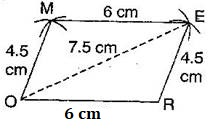(iv) Given: BE = 4.5 cm, ET = 6 cm
To construct: A rhombus BEST
Steps of construction:
(a) Draw TE = 6 cm and bisect it into two equal parts.
(b) Draw up and down perpendiculars to TE.
(c) Draw two arcs of 4.5 cm taking E and T as centres, which intersect at S.
(d) Again draw two arcs of 4.5 cm taking E and T as centres, which intersects at B.
(e) Join TS, ES, BT and EB.
This is the required rhombus BEST.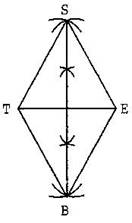Pg No. 63

Exercise 4.2

LI = 4 cm, IF = 3 cm, TL = 2.5 cm, LF = 4.5 cm, IT = 4 cm

OL = 7.5 cm, GL = 6 cm, GD = 6 cm, LD = 5 cm, OD = 10 cm

(iii) Rhombus BEND
BN = 5.6 cm, DE = 6.5 cm

Solution

(i) Given: LI = 4 cm, IF = 3 cm, TL = 2.5 cm, LF = 4.5 cm, IT = 4 cm
Steps of construction:
(a) Draw a line segment LI = 4 cm.
(b) Taking radius 4.5 cm, draw an arc taking L as centre.
(c) Draw an arc of 3 cm taking I as centre which intersects the first arc at F.
(d) Join FI and FL.
(e) Draw another arc of radius 2.5 cm taking L as centre and 4 cm taking I as centre which intersect at T.
(f) Join TF, Tl and TI.
This is the required quadrilateral LIFT.(ii) Given: OL = 7.5 cm, GL = 6 cm, GD = 6 cm, LD = 5 cm, OD = 10 cm
Steps of construction:
(a) Draw a line segment OL = 7.5 cm.
(b) Draw an arc of radius 5 cm taking L as centre and another arc of radius 10 cm taking O as centre which intersect the first arc at point D.
(c) Join LD and OD.
(d) Draw an arc of radius 6 cm from D and draw another arc of radius 6 cm taking L as centre, which intersects at G.
(e) Join GD and GO.
This is the required quadrilateral GOLD.(iii) Given: BN = 5.6 cm, DE = 6.5 cm
To construct: A rhombus BEND
Steps of construction:
(a) Draw DE = 6.5 cm.
(b) Draw perpendicular bisector of line segment DE.
(c) Draw two arcs of radius 2.8 cm from intersection point O, which intersects the perpendicular bisector at B and N.
(d) Join BE, BD as well as ND and NE.
This is the required rhombus BEND.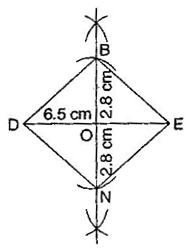Pg No. 64

Exercise 4.3

MO = 6 cm, OR = 4.5 cm, ∠M = 60°, ∠O = 105°, ∠R = 105°

PL = 4 cm, LA = 6.5 cm, ∠P = 90°, ∠A = 110°, ∠N = 85°

(iii) Parallelogram HEAR
HE = 5 cm, EA = 6 cm, ∠R = 85°

(iv) Rectangle OKAY
OK = 7 cm, KA = 5 cm

Solution

(i) Given: MO = 6 cm, OR = 4.5 cm,
∠M = 60°, ∠O = 105°, ∠R = 105°
Steps of construction:
(a) Draw a line segment MO = 6 cm.
(b) Construct ∠R = 105° and taking radius 4.5 cm, draw an arc taking O as centre, which intersects at R.
(c) Also construct an angle 105° at R and produce the side RE.
(d) Construct another angle of 60° at point M and produce the side ME. Both sides ME and RE intersect at E.
This is the required quadrilateral MORE.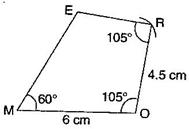(ii) Given: PL = 4 cm, LA = 6.5 cm,
∠P = 90°, ∠A = 110°, ∠N = 85°
To find: ∠L = 360° - (90° + 85° + 110°) = 360° - 285° = 75°
Steps of construction:
(a) Draw a line segment PL = 4 cm.
(b) Construct angle of 90° at P and produce the side PN.
(c) Construct angle of 75° at L and with L as centre, draw an arc of radius 6 cm, which intersects at A.
(d) Construct ∠A = 110° at A and produce the side AN which intersects PN at N.
This is the required quadrilateral PLAN.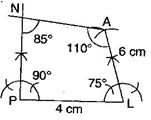(iii) Given: HE = 5 cm, EA = 6 cm, ∠R = 85°
To construct: A parallelogram HEAR
To find:  ∠H = 180° - 85° = 95° [Sum of adjacent angle of ||gm is 180°]
Steps of construction:
(a) Draw a line segment HE = 5 cm.
(b) Construct ∠H = 95° and draw an arc of radius 6 cm with centre H. It intersects AR at R.
(c) Join RH.
(d) Draw ∠R = ∠E = 85° and draw an arc of radius 6 cm with E as a centre which intersects RA at A.
(e) Join RA.
This is the required parallelogram HEAR.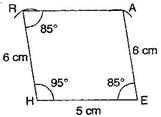(iv) Given: OK = 7 cm, KA = 5 cm
To construct: A rectangle OKAY
Steps of construction:
(a)  Draw a line segment OK = 7 cm.
(b) Construct angle 90° at both points O and K and produce these sides.
(c) Draw two arcs of radius 5 cm from points O and K respectively. These arcs intersect at Y and A.
(d) Join YA.
This is the required rectangle OKAY.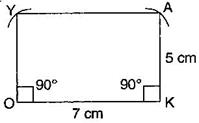Pg No. 67

Exercise 4.4

DE = 4 cm, EA = 5 cm, AR = 4.5 cm, ∠E = 60°, ∠A = 90°

TR = 3.5 cm, RU = 3 cm, UE = 4 cm, ∠R = 75°, ∠U = 120°

Solution

(i) Given: DE = 4 cm, EA = 5 cm, AR = 4.5 cm, ∠E = 60°, ∠A = 90°
Steps of construction:
(a) Draw a line segment DE = 4 cm.
(b) At point E, construct an angle of 60°.
(c) Taking radius 5 cm, draw an arc from point E which intersects at A.
(d) Construct ∠A = 90°, draw an arc of radius 4.5 cm with centre A which intersect at R.
(e) Join RD.
This is the required quadrilateral DEAR.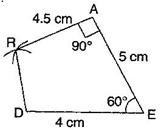(ii) Given: TR = 3.5 cm, RU = 3 cm, UE = 4 cm, ∠R = 75°, ∠U = 120°
Steps of construction:
(a) Draw a line segment TR = 3.5 cm.
(b) Construct an angle 75° at R and draw an arc of radius 3 cm with R as centre, which intersects at U.
(c) Construct an angle of 120° at U and produce the side UE.
(d) Draw an arc of radius 4 cm with U as centre.
(e) Join UE and TE.
This is the required quadrilateral TRUE.Pg No. 68

Exercise 4.5

Draw the following:
1. The square READ with RE = 5.1 cm.

Solution

Given: RE = 5.1 cm
Steps of construction:
(i)  Draw a line segment RE = 5.1 cm.
(ii)   At point E, construct an angle of  90o and draw an arc of radius 5.1 cm, mark the intesection of line and arc as point A.
(iii) From point R, draw an arc of radius 5.1 cm and from point A, draw another arc of radius 5.1 cm, mark the intersection of the two arcs as point D.
This is the required square READ.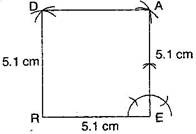2. A rhombus whose diagonals are 5.2 cm and 6.4 cm.

Solution

Given:  Diagonals of a rhombus
AC = 5.2 cm and BD = 6.4 cm.
To construct: A rhombus ABCD.
Steps of construction:
(i) Draw AC = 5.2 cm and draw a perpendicular bisector on AC.
(ii) From the midpoint O, draw two arcs of radius 3.2 cm cutting the perpendicular bisector on both sides.
(iii) Mark the points of intersection of arcs and perpendicular bisector as B and D.
(iv) Join AB, BC, CD and DA.
This is the required rhombus ABCD.3. A rectangle with adjacent sides of length 5 cm and 4 cm.

Solution

Given:  MN = 5 cm and MP = 4 cm.
To construct: A rectangle MNOP
Steps of construction:
(i) Draw a segment MN = 5 cm.
(ii) At points M and N, draw perpendiculars of lengths 4 cm and produce them.
(iii) Taking centres M and N, draw two arcs of 4 cm each, which intersect P and Q respectively.
(iv) Join side PO.
This is the required rectangle MNOP.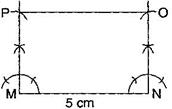4. A parallelogram OKAY where OK = 5.5 cm and KA = 4.2 cm.

Solution

OK = 5.5 cm and KA = 4.2 cm.
To construct: A parallelogram OKAY.
Steps of construction:
(i) Draw a line segment OK = 5.5 cm.
(ii) Draw an angle of 90o at K and draw an arc of radius KA = 4.2 cm, which intersects at point A.
(iii) Draw another arc of radius AY = 5.5 cm and at point O, draw another arc of radius 4.2 cm which intersect at Y.
(iv) Join AY and OY.
This is the required parallelogram OKAY.## NCERT Solutions for Class 8 Maths Chapter 4 Practical Geometry

Chapter 4 NCERT Solutions help you in knowing the important concepts of the chapter and helpful in getting good marks in the examinations. In Chapter 4 Class 8 Maths, we shall learn how to construct a unique quadrilateral given the following measurements:
• When four sides and one diagonal are given.
• When two diagonals and three sides are given.
• When two adjacent sides and three angles are given.
• When three sides and two included angles are given.
• When other special properties are known.

We have provided exercisewise NCERT Solutions for Class 8 Maths that will help in clearing the basic concepts and always find the question that you're looking for.

Subject matter experts of Studyrankers have provided detailed solutions of every question step by step so a student can easily clear their doubts and study efficiently.

### NCERT Solutions for Class 8 Maths Chapters:

 Chapter 1 Rational Numbers Chapter 2 Linear Equations in Variable Chapter 3 Understanding Quadrilaterals Chapter 5 Data Handling Chapter 6 Square and Square Roots Chapter 7 Cube and Cube Roots Chapter 8 Comparing Quantities Chapter 9 Algebraic Expressions and Identities Chapter 10 Visualizing Solid Shapes Chapter 11 Mensuration Chapter 12 Exponents and Powers Chapter 13 Direct and Inverse Proportions Chapter 14 Factorization Chapter 15 Introduction to Graphs Chapter 16 Playing with Numbers

FAQ on Chapter 4 Practical Geometry

#### How many exercises in Chapter 4 Practical Geometry?

There are total 5 exercises in the the Chapter 4 Class 8 Maths NCERT Solutions which will improve your problem solving skills and enhance your knowledge. The concepts learned in this chapter will going to help you in higher classes a lot.

#### To construct a line segment of a given length, what type of instruments are needed?

Ruler and Compass are used to construct a line segment.

#### What are the benefits of NCERT Solutions for Chapter 4 Practical Geometry Class 8 NCERT Solutions?

These NCERT Solutions for Class 8 are updated as per the latest guidelines introduced by CBSE thus one can improve their marks in the exams. Also, they can understand the concepts behind the questions efficiently solve the problems.

#### How can I download PDF of Chapter 4 Practical Geometry NCERT Solutions Class 8 Maths?

You can download the Chapter 4 NCERT Solutions PDF on this page and practice these whenever in need. This will develop your skills and let you learn variety of topics.